# DOS功能调用一览表

DOS2.10使用了20H-27H,其功能定义为:

 中断 功能 入口参数 出口参数 INT20 程序正常退出 CS=PSP段地址 INT21 系统功能调用 AH=功能号 INT22 程序结束处理 INT23 Ctrl-Break处理 AL=0(忽略) INT24 严重错误处理 AL=驱动器号 AL=1(重试) AL=2(通过INT 23H终止) Cy=1出错 INT25 绝对磁盘读 CX=读入扇区数 DX=起始逻辑扇区数 DS:BX=缓冲区地址 AL=驱动器号 Cy=0正确 INT26 绝对磁盘写 CX=写盘扇区数 DX=起始逻辑扇区数 DS:BX=缓冲区地址 INT27 驻留退出 CS=PSP段地址 DX=程序末地址+1

 调用号 功能 入口参数 出口参数 00H 程序终止 CS=PSP段地址 01H 键盘输入字符 AL=输入的字符 02H 显示输出 DL=显示的字符 03H 串行设备输入 AL=输入的字符 04H 串行设备输出 DL=输出的字符 05H 打印输出 DL=输出的字符 06H 直接控制台I/O DL=0FFH(输入请求) DL=字符(输出请求) AL=输入的字符 07H 直接控制台I/O (不显示输入) AL=输入的字符 08H 键盘输入字符(无回显) AL=输入的字符 09H 显示字符串 DS:DX=缓冲区首址 0AH 输入字符串 DS:DX=缓冲区首址 0BH 检查标准输入状态 AL=00无按键 AL=0FFH有按键 0CH 清除输入缓冲区并执行指定的标准输入功能 AL=功能号 (01/06/07/08/0AH) DS:DX=缓冲区(0AH功能) AL=输入的数据 (功能01/06/07/08) 0DH 初始化磁盘状态 0EH 选择缺省的驱动器 DL=驱动器号(0=A,1=B..) AL=逻辑驱动器数 0FH 打开文件 DS:DX=未打开的FCB首址 AL=00成功,0FFH失败 10H 关闭文件 DS:DX=打开的FCB首址 AL=00成功,0FFH失败 11H 查找第一匹配目录 DS:DX=未打开的FCB首址 AL=00成功,0FFH失败 12H 查找下一匹配目录 DS:DX=未打开的FCB首址 AL=00成功,0FFH失败 13H 删除文件 DS:DX=未打开的FCB首址 AL=00成功,0FFH失败 14H 顺序读 DS:DX=打开的FCB首址 AL=00成功,01文件结束 02缓冲区太小 03缓冲区不满 15H 顺序写 DS:DX=打开的FCB首址 AL=00成功,01盘满 02缓冲区太小 16H 创建文件 DS:DX=未打开的FCB首址 AL=00成功 0FFH目录区满 17H 文件换名 DS:DX=被修改的FCB首址 AL=00成功,0FFH未找 到目录项或文件重名 *18H 保留未用 19H 取缺省驱动器号 AL=驱动器号(0=A,1=B..) 1AH 设置磁盘缓冲区DTA DS:DX=磁盘缓冲区首址 *1BH 取缺省驱动器的磁盘格 式信息 AL=每簇的扇区数 CX=每扇区的字节数 DX=数据区总簇数-1 DS:BX=介质描述字节 *1CH 取指定驱动器的磁盘格 式信息 DL=驱动器号(0=缺省, 1=A..) AL=每簇的扇区数 CX=每扇区的字节数 DX=数据区总簇数-1 DS:BX=介质描述字节 *1DH 保留未用 *1EH 保留未用 *1FH 取缺省驱动器的DPB DS:BX=DPB首址 *20H 保留未用 21H 随机读一个记录 DS:DX=打开的FCB首址 AL=00成功,01文件结束 02缓冲区太小 03缓冲区不满 22H 随机写一个记录 DS:DX=打开的FCB首址 AL=00成功,01盘满 02缓冲区太小 23H 取文件大小 DS:DX=未打开的FCB首址 AL=00成功,0FFH失败 24H 设置随机记录号 DS:DX=打开的FCB首址 25H 设置中断向量 AL=中断号 DS:DX=中断程序入口 *26H 创建新的PSP DS:DX=新的PSP段地址 27H 随机读若干记录 DS:DX=打开的FCB首址 CX=要读入的记录数 AL=00成功,01文件结束 AL=02缓冲区太小 AL=03缓冲区不满 CX=读入的块数 28H 随机写若干记录 DS:DX=打开的FCB首址 CX=要写入的记录数 AL=00成功,01盘满 AL=02缓冲区太小 AL=03缓冲区不满 CX=已写的块数 29H 分析文件名 AL=分析控制标记 DS:SI=要分析的字符串 ES:DI=未打开的FCB首址 AL=00未通配符 01有通配符 0FFH驱动器字母无效 ES:DI=未打开的FCB 2AH 取系统日期 CX=年(1980-2099) DH=月,DL=日,AL=星期 (0=星期日) 2BH 置系统日期 CX=年,DH=月,DL=日 AL=00成功,0FFH失败 2CH 取系统时间 CH=时(0-23),CL=分,DH= 秒,DL=百分之几秒 2DH 置系统时间 CX=时,分DX=秒,百分秒 AL=00成功,0FFH失败 2EH 设置/复位校验开关 AL=0关闭,1打开 2FH 取磁盘传输地址DTA ES:BX=DTA首地址 30H 取DOS版本 AL,AH=DOS主、次版本 31H 结束并驻留 AL=返回码,DX=内存大小 *32H 取指定驱动器的DPB DS:BX=DPB首址 33H 取或置Ctrl-Break标志 AL=0:取,1:置,DL=标志 DL=标志(取功能)0:关 1:开 *34H 取DOS中断标志 ES:BX=DOS中断标志 35H 取中断向量地址 AL=中断号 ES:BX=中断程序入口 36H 取磁盘的自由空间 DL=驱动器号(0=缺省,1=A AX=FF驱动器无效 其它每簇扇区数 BX=自由簇数 CX=每扇区字节数 BX=文件区所占簇数 *37H 取/置参数分隔符 取/置设备名许可标记 AL=0:取分隔符,1:置分隔 符,DL=分隔符 2:取许可标记 3:置许可标记,DL=许 可标记 DL=分隔符(功能0) DL=许可标记(功能2) 38H 取国家信息 AL=0,DS:DX=缓冲区首址 39H 创建子目录 DS:DX=路径字符串 CF=0成功,1失败,AX=错 误码 3AH 删除子目录 DS:DX=路径字符串 CF=0成功,1失败,AX=错 误码 3BH 设置子目录 DS:DX=路径字符串 CF=0成功,1失败,AX=错 误码 3CH 创建文件 DS:DX=带路径的文件名 CX=属性1-只读2-隐蔽 4-系统 CF=0成功,AX=文件号 CF=1失败,AX=错误码 3DH 打开文件 DS:DX=带路径的文件名 AL=方式0-读1-写2-读 写 CF=0成功,AX=文件号 CF=1失败,AX=错误码 3EH 关闭文件 BX=文件号 CF=0成功 CF=1失败,AX=错误码 3FH 读文件或设备 BX=文件号 CX=字节数 CF=0成功 DX:AX=新的指针位置 40H 写文件或设备 DS:DX=缓冲区首址 CF=1失败,AX=错误码 41H 删除文件 DS:DX=带路径的文件名 CF=0成功,1失败,AX=错 误码 42H 移动文件指针 AL=方式0-正向1-相对 2-反向 BX=文件号,CX:DX=移动的 位移量 CF=0成功,DX:AX=新的文 件指针 CF=1失败,AX=错误码 43H 取/置文件属性 AL=0:取1:置,CX=新属性 DS:DX=带路径的文件名 CX=属性(功能0)1-只读 2-隐蔽4-系统20H-归档 44H 设备输入/输出控制: 设置/取得与打开设备 的句柄相关联信息,或 发送/接收控制字符串 至设备句柄 AL=0/1取/置设备信息 2/3读/写设备控制通道 4/5同功能2/3 6/7取输入/输出状态 BX=句柄(功能0-3,6-7) BL=驱动器号(功能4-5) CX=字节数(功能2-5) DS:DX=缓冲区(功能2-5) CF=0成功 DX=设备信息(功能0) AL=状态(功能6/7) 0未准备,1准备 AX=传送的字节数(功能2- 5) 45H 复制文件号(对于一个 打开的文件返回一个新 的文件号) BX=文件号 CF=0成功,AX=新文件号 CF=1失败,AX=错误码 46H 强行复制文件号 BX=现存的文件号,CX=第2 文件号 CF=0成功,1失败 AX=错误码 47H 取当前目录 DL=驱动器号 DS:SI=缓冲区首址 CF=0成功,1失败 AX=错误码 48H 分配内存 BX=所需的内存节数 CF=0成功,AX=分配的段 数,CF=1失败,AX=错误码 BX=最大可用块大小 49H 释放内存 ES=释放块的段值 CF=1失败,AX=错误码 4AH 修改分配内存 ES=修改块的段值 BX=新长度(以节为单位) CF=1失败,AX=错误码 BX=最大可用块大小 4BH 装载程序 运行程序 AL=0装载并运行 1获得执行信息 3装载但不运行 DS:DX=带路径的文件名 ES:BX=装载用的参数块 CF=1失败,AX=错误码 4CH 带返回码的结束 AL=进程返回码 4DH 取由31H/4CH带回的返 回码 AL=进程返回码 AH=类型码,0-正常结束 1-由Ctrl-Break结束 2-由严重设备错误而结束 3-由调用31H而结束 4EH 查找第一个匹配项 DS:DX=带路径的文件名 CX=属性 CF=1失败,AX=错误码 4FH 查找下一个匹配项 CF=1失败,AX=错误码 *50H 建立当前的PSP段地址 BX=PSP段地址 *51H 读当前的PSP段地址 BX=PSP段地址 *52H 取DOS系统数据区首址 ES:BX=DOS数据区首址 *53H 为块设备建立DPB DS:SI=BPB,ES:DI=DPB 54H 取校验开关设定值 AL=标志值(0:关,1:开) *55H 由当前PSP建立新PSP DX=PSP段地址 56H 文件换名 DS:DX=带路径的旧文件名 ES:DI=带路径的新文件名 CF=1失败,AX=错误码 57H 取/置文件时间及日期 AL=0/1取/置,BX=文件号 CX=时间,DX=日期 CF=0成功,CX=时间,DX= 日期

CF=1失败,AX=错误码

 错误码 错误类型 错误码 错误类型 01H 无效的功能号 0AH 不正确的环境 02H 文件未找到 0BH 不正确的格式 03H 路径未找到 0CH 无效的存取代码 04H 打开的文件太多 0DH 无效的数据 05H 拒绝存取 0EH 保留 06H 非法的文件号 0FH 指定的驱动器无效 07H 内存控制块破坏 10H 试图删除当前目录 08H 没有足够的内存空间 11H 非同一设备 09H 无效的内存块地址 12H 没有更多的文件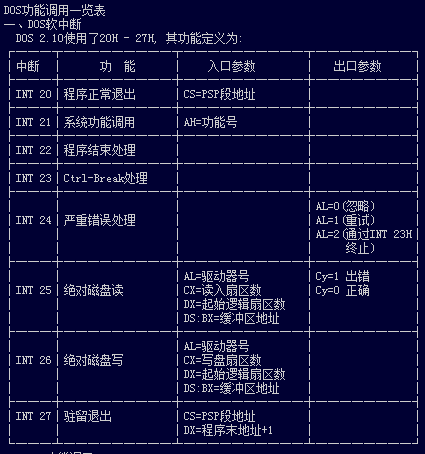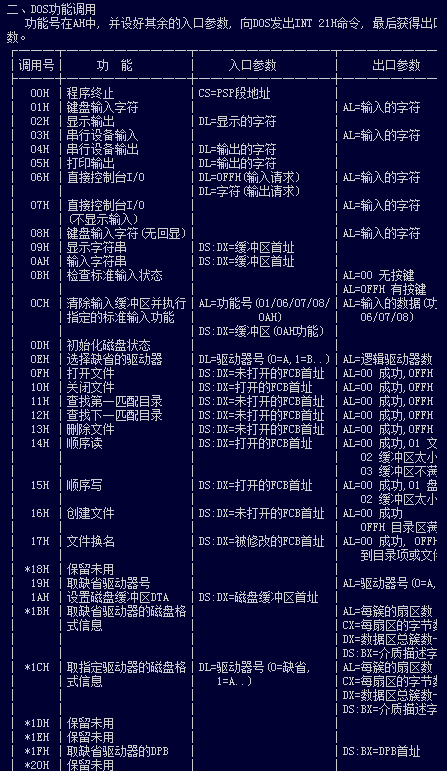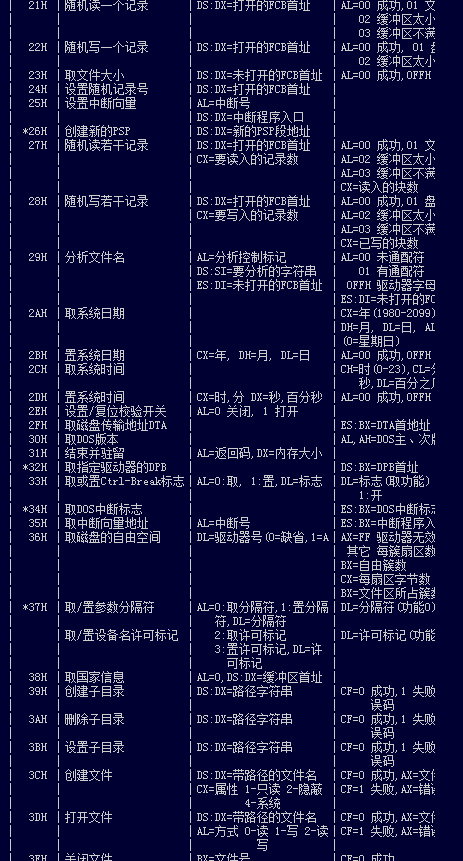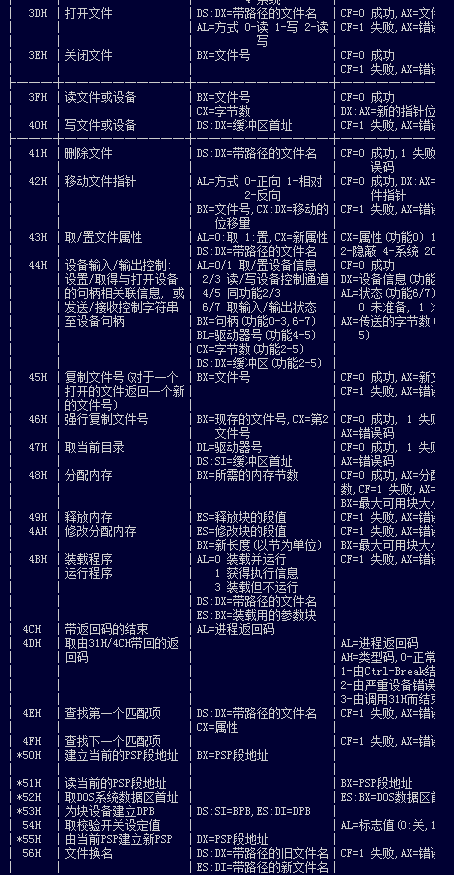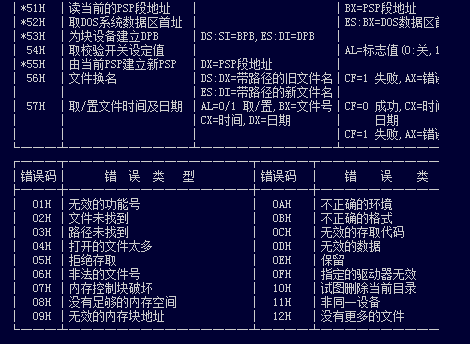04-221705

#### DOS功能调用( INT 21 )一览表

08-105万+

#### DOS系统功能调用表(INT 21H)

12-03369

#### DOS命令详细一览表

12-031041

#### INT 21H 功能调用

09-08379

#### 电脑DOS命令一览表

10-133108

#### 中断向量地址一览表

04-171613

#### 错误代码说明一览表

09-2710

#### 系统进程一览表

03-126278

#### 文件后缀名大全

03-101980

#### 命令提示符常用命令一览表

11-106294

#### windows错误代码一览表

03-26939

#### DOS命令大全

06-2128

#### windows xp进程最佳配置

03-044108

#### 常用端口号信息一览表

06-1511

#### 计算机常用端口一览表

04-1462万+

#### 在中国程序员是青春饭吗？

03-1316万+

#### 程序员请照顾好自己，周末病魔差点一套带走我。

03-123222

#### C++(数据结构与算法)78:---分而治之

02-281万+

#### springboot+jwt实现token登陆权限认证

03-0114万+

#### 技术大佬：我去，你写的 switch 语句也太老土了吧©️2019 CSDN 皮肤主题: 1024 设计师: 上身试试点击重新获取扫码支付